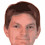## Sunday, 5 August 2012

### RGB/HCY in HLSL

The HCY colour space is a tractable hue/chroma/luminance scheme developed by Kuzma Shapran. It is ideal for pixel shaders, being only slightly more expensive that the HSV and HSL schemes. However, it tries to be more "meaningful" in terms of human perception.

The three components are:
1. Hue (H) computed in the same manner as HSV and HSL;
2. Chroma (C) computed as the scaled difference between the maximum unweighted RGB component and the minimum unweighted RGB component; and
3. Luminance (Y) computed as the weighted sum of RGB components.
Note that the chroma is post-scaled so that the maximum weighted luminance for this hue is always one.

The HLSL conversions are as follows:
```// The weights of RGB contributions to luminance.
// Should sum to unity.
float3 HCYwts = float3(0.299, 0.587, 0.114);

float3 HUEtoRGB(in float H)
{
float R = abs(H * 6 - 3) - 1;
float G = 2 - abs(H * 6 - 2);
float B = 2 - abs(H * 6 - 4);
return saturate(float3(R,G,B));
}

float RGBCVtoHUE(in float3 RGB, in float C, in float V)
{
float3 Delta = (V - RGB) / C;
Delta.rgb -= Delta.brg;
Delta.rgb += float3(2,4,6);
// NOTE 1
Delta.brg = step(V, RGB) * Delta.brg;
float H;
#if NO_ASM
H = max(Delta.r, max(Delta.g, Delta.b));
#else
float4 Delta4 = Delta.rgbr;
asm { max4 H, Delta4 };
#endif
return frac(H / 6);
}

float3 RGBtoHCY(in float3 RGB)
{
float3 HCY = 0;
float U, V;
#if NO_ASM
U = -min(RGB.r, min(RGB.g, RGB.b));
V = max(RGB.r, max(RGB.g, RGB.b));
#else
float4 RGB4 = RGB.rgbr;
asm { max4 U, -RGB4 };
asm { max4 V, RGB4 };
#endif
HCY.y = V + U;
HCY.z = dot(RGB, HCYwts);
if (HCY.y != 0)
{
HCY.x = RGBCVtoHUE(RGB, HCY.y, V);
float Z = dot(HUEtoRGB(HCY.x), HCYwts);
if (HCY.z > Z)
{
HCY.z = 1 - HCY.z;
Z = 1 - Z;
}
HCY.y *= Z / HCY.z;
}
return HCY;
}

float3 HCYtoRGB(in float3 HCY)
{
float RGB = HUEtoRGB(HCY.x);
float Z = dot(RGB, HCYwts);
if (HCY.z < Z)
{
HCY.y *= HCY.z / Z;
}
else if (Z < 1)
{
HCY.y *= (1 - HCY.z) / (1 - Z);
}
return (RGB - Z) * HCY.y + HCY.z;
}
```

I've folded the code into my web page on such conversions here.

1.Hi Ian,

Thanks for sharing this very useful color conversion code. Is this code free to use? does it carry any specific license?

Thanks!

2.This comment has been removed by a blog administrator.

3.@joe : Yes, feel free to use the code. The original algorithms on which it is based (https://code.google.com/archive/p/colour-space-viewer/) appear to be GPLv3.